# Python Loops | Lesson 3 Data Analytics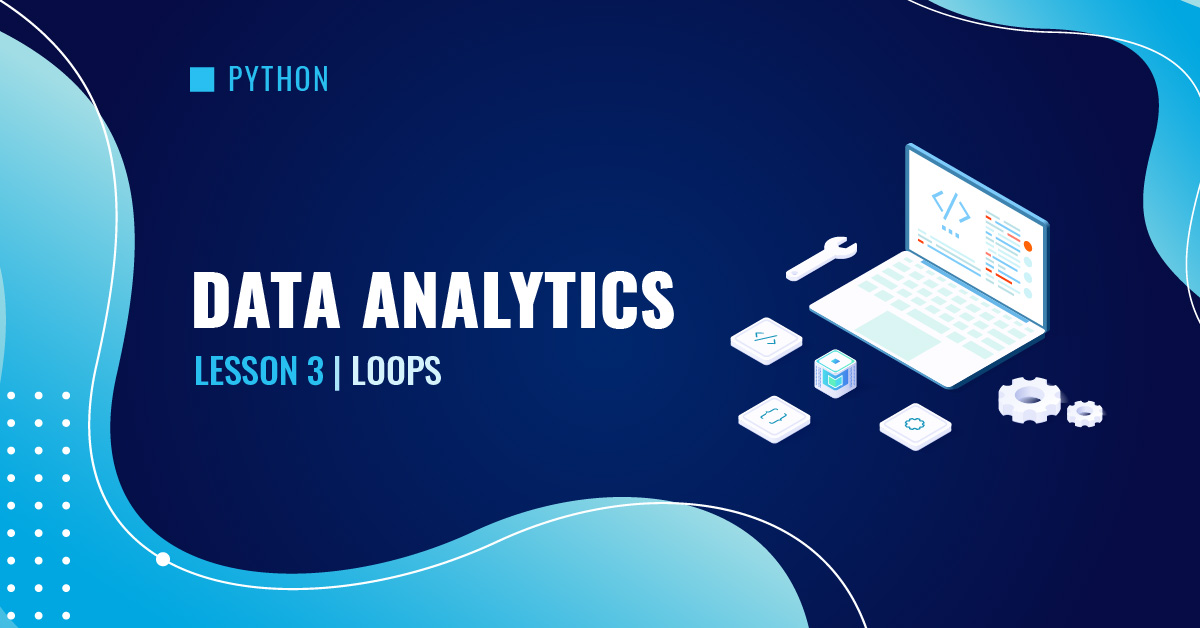## Lesson 3: Loops

Loops in the context of programming refer to repetitive actions until a specified condition is met. For example, to create a list containing the first 10 integers, instead of using 10 lines of code to append the integers, a loop can simplify the code to as little as 1 line.

### While Loops

While loops iterate as long as a logical test is True. Consider the following lines of code: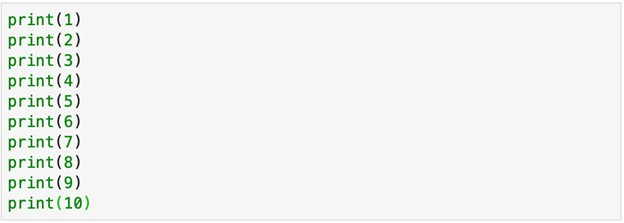This approach is not efficient and effective. If there are tens or even hundreds of numbers to be printed, we would need to type hundreds of lines of code.

The above approach can be replaced by a While loop: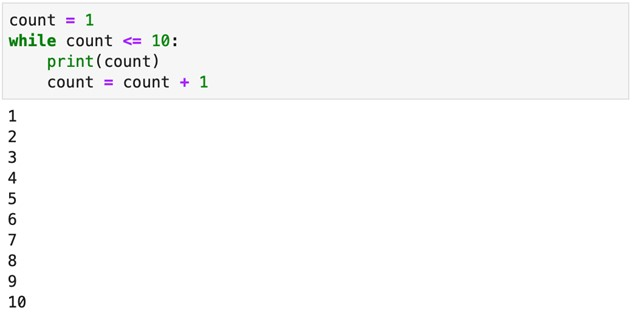In the above example, the variable count is initially assigned the value of 1. The While loop first tests if the variable count is less than or equal to 10. If it is less than or equal to 10, the operations in the loop are executed; otherwise, the iterations stop.

• The 1st iteration: count = 1, so print the value of count (1),  then update the value of count to 2 and repeat.
• The 2nd iteration: count = 2,  so print the value of count (2), then update the value of count to 3 and  repeat.
• The 3rd iteration: count = 3, so print the value of count (3), then update the value of count to 4 and repeat.

The 10th iteration: count = 10, so print the value of count (10), then update the value of count to 11 and repeat.

On the 11th iteration, because the value of the variable count (11) is no longer less than or equal to 10, the loop is broken and no further iterations are performed.

A While loop can also be used to access the values in a sequence – string, list, tuple, and dictionary.

For example, to print out the characters in the string “AICPA”, instead of typing 5 lines to print, a While loop can be used.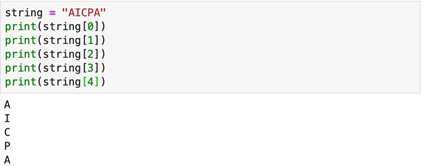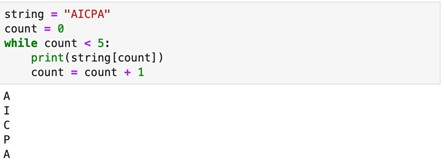You may have noticed that the While loop in the example above requires counting the number of characters in the string “AICPA” in advance. Because the length of the string is 5, the While loop needs to iterate 5 times.

To simplify the process, the len ( ) function, which returns the length of a sequence, can be used to find the number of iterations needed. Thus, the code can be revised as: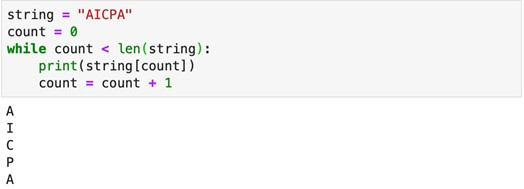Similarly, to access the elements in a list, the combination of a While loop and the len ( ) function can be used.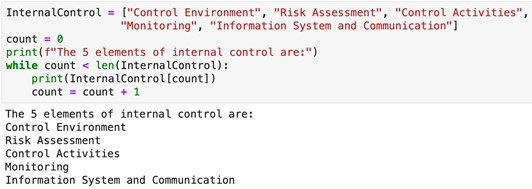The above examples use the expression count = count + 1 to update the value in the variable count in each loop. This expression can be simplified using the increment or decrement operators listed in the table below.

OperatorExampleMeaning
+=count += 1count = count + 1
-=count -= 1count = count – 1
*=count *= 2count = count x 2
/=count /= 2count = count ÷ 2

Therefore, the previous example can be revised as: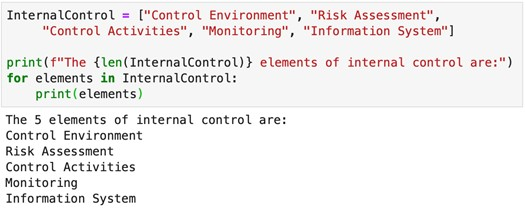### For Loops

For loops directly iterate through a sequence. While the While loop uses a counter and the expression count += 1 to denote each iteration, a For loop uses the in operator to access the elements in a sequence or denote each iteration.

To print the elements of internal control, the following For loop can be used:The For Loop in the above example has the following structure: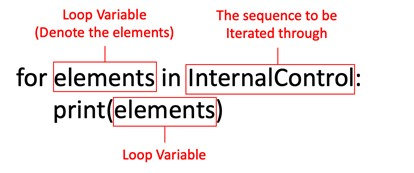In each iteration, the For loop accesses an element of the sequence (e.g., “Control Environment”, “Risk Assessment”, etc.) and performs the operations. The loop is broken when all the elements are iterated through.

• The 1st loop: for the first element “Control Environment,” print the element, then go to Next element.
• The 2nd loop: for the second element “Risk Assessment,” print the element, then go to Next element.
• The 5th loop: for the last element “Information System,” print the element, then stop.

Similarly, to print each character in the string “AICPA,” the following For loop can be used: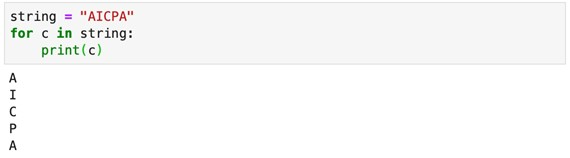In this example, the loop variable named “c” refers to the characters in the string “AICPA.” In each iteration, the loop variable is printed.

Besides referencing the elements of a sequence by a loop variable, the elements can be accessed by their indices. For example, the following For loop prints the elements in the list InternalControl: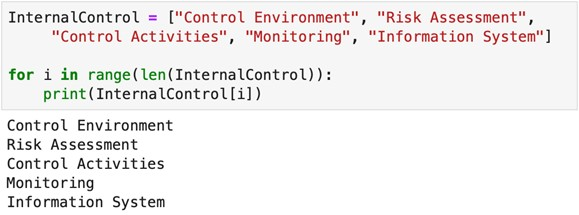The above For loop creates a range of numbers using the range ( ) function. In every iteration, the numbers are iterated through.

### Range ( ) Function

The range ( ) function can be used to quickly create a sequence of numbers for a For loop to iterate through.
Below is the syntax of  the range ( )  function: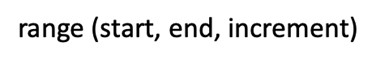• Start: the number from which the range starts
• End: the number (exclusive) to which the range ends
• Increment: the increment (defaulted as 1) between the starting number and the ending number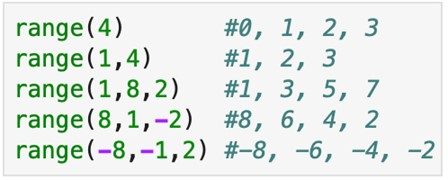Therefore, a sequence of the first 10 integers can be created using range(1,11).  Because the range ( )  function by default starts from zero, the range between 1 and 11(exclusive) must be specified.

Therefore, the expression means the following: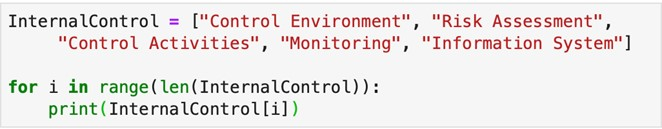1. len(InternalControl) returns the length of the list InternalControl, which is 5.
2. range(len(InternalControl)) creates a number sequence range(5), which is 0,1,2,3,4.
3. The For loop iterates through the number sequence and prints the indexed element:
• The 1st loop: for the number 0, print InternalControl, then go to Next number.
• The 2nd loop: for the number 1, print InternalControl, then go to Next number.
• The 5th loop: for the number 4, print InternalControl, then stop.

If the elements of a sequence are referenced by a loop variable, all the elements must be iterated through. If the elements of a sequence are accessed by their indices, operations on a slice of the sequence can be performed. The following example prints only the second to the fourth elements of the list InternalControl.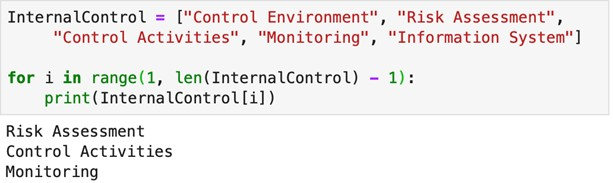### Break and Continue

Break
Loops continue to run as long as their criterion is met, but occasionally you may find that you  want to stop iterating part-way through. For these occasions, we have the break keyword.

For example, suppose we want to print the numbers in an sequence up until the first one above some threshold: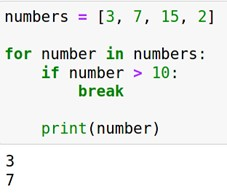For each item in the sequence we use the if statement to check if that item meets our threshold condition. If it does, we break the loop so we don’t print out any other numbers in the sequence.

Continue
Sometimes we may want to skip some iterations in a loop. The continue function skips the current block and proceeds to the next iteration.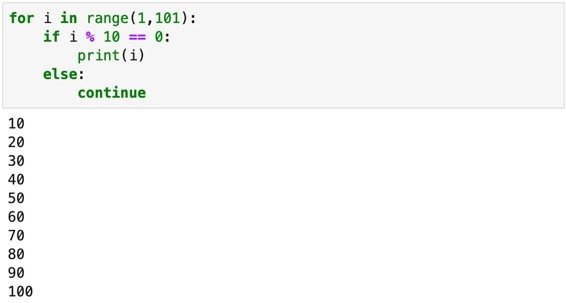The above For loop iterates through the number range between 1 and 100. If the number iterated through is a multiple of 10, it is printed; otherwise, the iteration is skipped.

List Comprehension
Consider the following code: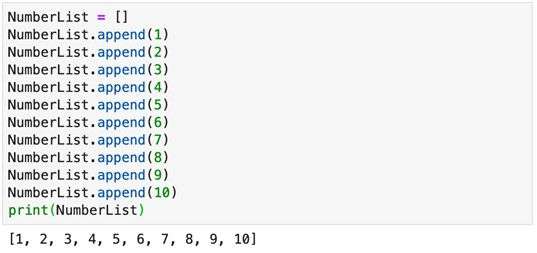An empty list is first created. Without using a loop, creating a list of the first 10 integers requires 10 lines of code to append the numbers 1 through 10 one by one to the empty list.

Using a While loop, the above code can be simplified as: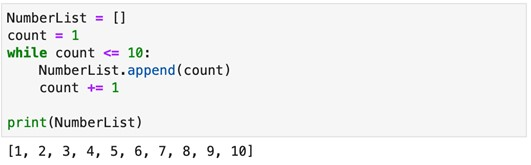Using a For loop, the above code can be simplified as: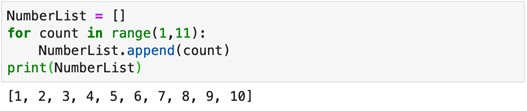To further simplify the creation of a list, a list comprehension can be used: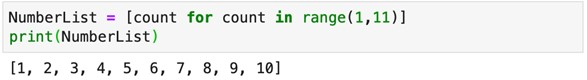To further simplify the creation of a list, a list comprehension can be used: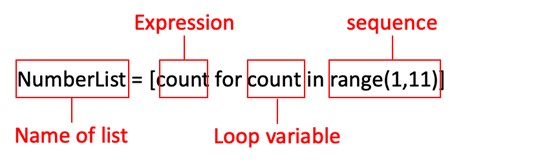To further simplify the creation of a list, a list comprehension can be used:

The expression determines any operations to the loop variable. In the following illustration, the numbers appended to the list are the squares of the loop variable’s count in the number range from 1 to 10.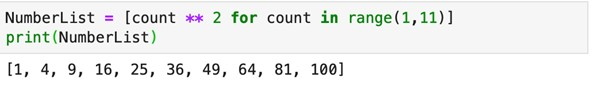Keeping in line with the “Zen of Python”, which states that “there should be one — and preferably only one — obvious way to do [something]”, each method of looping over data serves a different purpose. Knowing when to apply these methods is a useful skill that will let you write more natural, expressive code. In general, the order of preference would be:

1. List comprehension – Generating a new list.
2. for loop – Performing some action across each element of an existing list.
3. while loop – Performing some action repeatedly until a condition is met without knowing the explicit boundaries upfront.

Example: List Creation with Conditions

To create a list of even numbers between the range of 1 and 20, the following codes can be used:

While loop: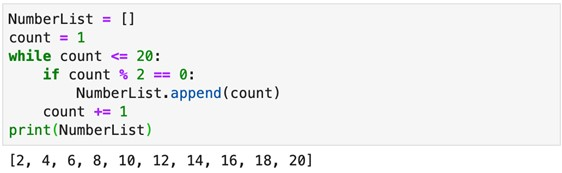For loop: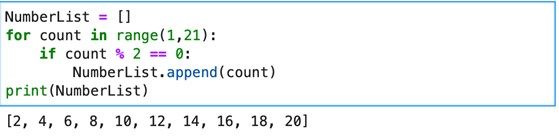List Comprehension: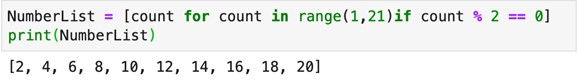The above list comprehension has the following syntax: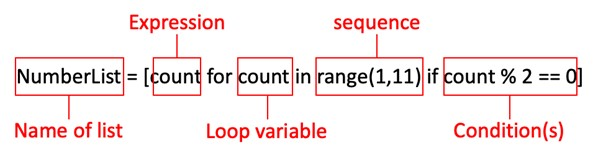Conditions can be added using the and, or, and not operators. The following example appends to the list the numbers between 1 and 20 that are even but not divisible by 4.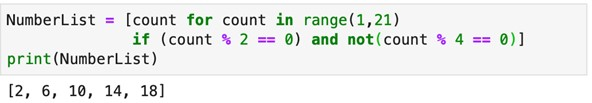Exercise:

1. Using a loop, calculate the average of the first 10 positive, non-zero integers.

While loop: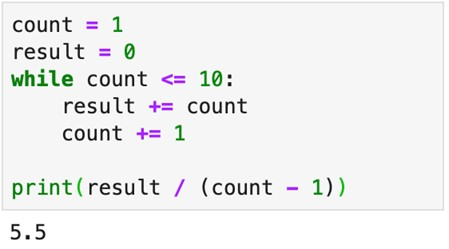For loop: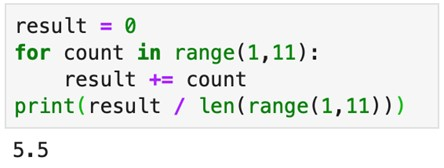2. Using a loop, calculate the value of the factorial of 5 [5! = 5 x 4 x 3 x 2 x 1].

While loop: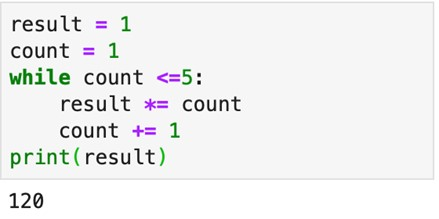For loop: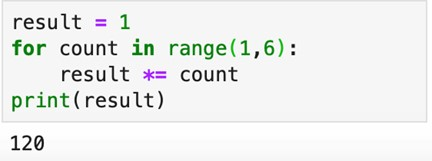3. Given two lists of the same length: ListA = [2, 4, 6, 8, 10] and ListB = [25, 30, 35, 40, 45]. Create a new list ListC to store the products of the corresponding elements of ListA and ListB.

While loop: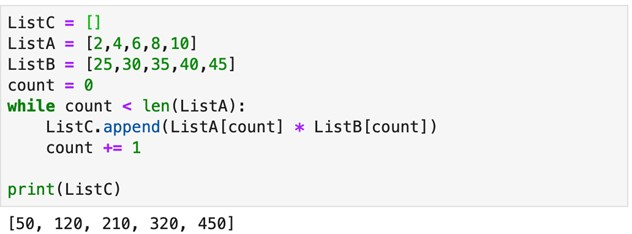For loop: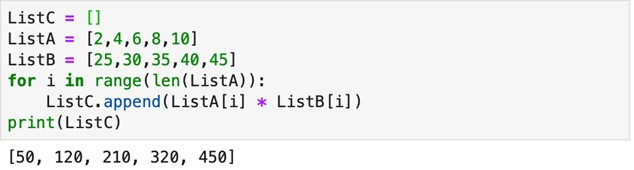List Comprehension: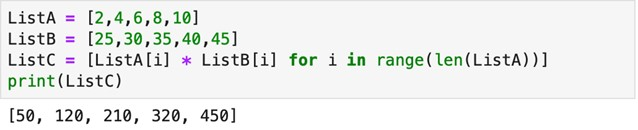### Learning Python Data Analytics with Gleim

At Gleim, we know learning data analytics is vital for future certified accountants. That is why we are offering this series of data analytics blogs and continually updating all of our review materials for CPA, CMA, and CIA with the necessary information you need to pass your exam the first time!

We’ll continue our weekly blog series. Check back regularly for all exam news and study tips!

Python Lessons
Python Basics
Conditional Statements
Loops
Functions and Modules
Numerical Python (NumPy)
Pandas Basics
Pandas Data Capture and Cleansing
Merging and Grouping
Manipulating Text and Datetime Data
Visualization
Web Scraping
Errors and Exceptions